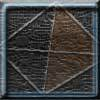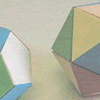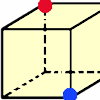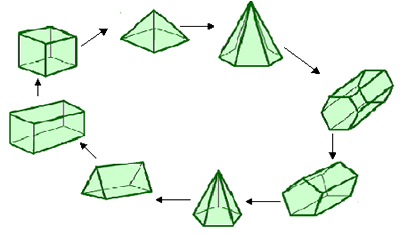You may also likeTetrahedron Faces

One face of a regular tetrahedron is painted blue and each of the remaining faces are painted using one of the colours red, green or yellow. How many different possibilities are there?Face Painting

You want to make each of the 5 Platonic solids and colour the faces so that, in every case, no two faces which meet along an edge have the same colour.Redblue

Investigate the number of paths you can take from one vertex to another in these 3D shapes. Is it possible to take an odd number and an even number of paths to the same vertex?

A Chain of Eight Polyhedra

Age 7 to 11Challenge Level

Many of you sent solutions to this problem, although none of you tried to find more than one way of making a chain. Wilson from Beecroft Primary School sent in a diagram which shows one chain very clearly. In fact Wilson noticed that this is a loop, not just a chain:Rachael, Chloe and Emilia from Ardingly College Junior School found a different chain:

Hexagonal prism
Hexagonal based pyramid
Pentagonal based pyramid
Pentagonal prism
Cuboid
Cube
Square based pyramid
Triangular prism

Zara, also from Ardingly College Junior School discovered another chain:

Triangular prism - Square based pyramid - cube - cuboid - pentagonal prism - hexagonal prism - hexagonal based pyramid - pentagonal based pyramid.
The links between these eight shapes are that between the triangular prism and square based pyramid are the triangle. Next the link is the square, then the square again. Between the cuboid and pentagonal prism is the rectangle. Next the link is rectangle again then the hexagon. Last of all the link is the triangle.

Do these make loops too?
Alex and Katie from Woodfall Junior described how the links worked in their loop:

Cube connects to the cuboid by a square.
Cuboid connects to the hexagonal prism by a rectangle.
Hexagonal prism connects to the hexagonal based pyramid by a hexagon.
Hexagonal based pyramid connects to the pentagonal based pyramid by an isoceles triangle.
Pentagonal based pyramid connects to the pentagonal prism by a pentagon.
Pentagonal prism connects to the triangular prism by a rectangle.
Triangular prism connects to the square based pyramid by an equilateral triangle.
Square based pyramid connects to the cube by a square.

Then we asked you how many different ways there are to make a loop.

Danny, aged 11, explained how he went about it:

I made a table of what types of faces each shape has, and then worked out from that which shapes can go next to each other.
 Square face Hexagon face Triangle face Pentagon face Rectangle face Cube Yes No No No No Hexagon-based pyramid No Yes Yes No No Pentagonal prism No No No Yes Yes Square-based pyramid Yes No Yes No No Hexagonal prism No Yes No No Yes Cuboid Yes No No No Yes Pentagon-based pyramid No No Yes Yes No Trianglar prism No No Yes No Yes

 Shape Goes with Cube Square-based pyramid, Cuboid Hexagon-based pyramid Hexagonal prism,Square-based pyramid,Pentagon-based pyramid,Trianglar prism Pentagonal prism Pentagon-based pyramid, Hexagonal prism, Cuboid, Trianglar prism Square-based pyramid Cube, Cuboid, Hexagon-based pyramid,Pentagon-based pyramid, Trianglar prism Hexagonal prism Hexagon-based pyramid, Pentagonal prism, Cuboid, Trianglar prism Cuboid Cube, Square-based pyramid,Pentagonal prism,Hexagonal prism,Trianglar prism Pentagon-based pyramid Pentagonal prism, Hexagon-based pyramid,Square-based pyramid,Trianglar prism Trianglar prism Hexagon-based pyramid ,Square-based pyramid,Pentagon-based pyramid,Pentagonal prism,Hexagonal prism,Cuboid

Then I saw that a cube can only go between the cuboid and the square-based pyramid, because they are the only two other shapes with square faces, so I put those three together. Then I saw which shapes could go next to the square-based pyramid, which are the cube, cuboid, hexagon-based pyramid, pentagon-based pyramid and triangular prism. But I'd already counted the cube and the cuboid, so I made a decision tree with three branches, one for the hexagon-based pyramid, one for the pentagon-based pyramid and one for the triangular prism.

I made branches from those using all the shapes they could go with that I hadn't used before, and continued until either the branch couldn't go any further or I'd counted all 8 shapes. I made 27 branches using all 8 shapes.

Then I had to see if they could join back to the cuboid again. Only the prisms could, because the other shapes had either been used to start off the chain or didn't have any faces the same as the cuboid. So I had 17 branches with all 8 shapes that could join back to the cuboid to make a loop.

Fantastic, Danny! Thank you!